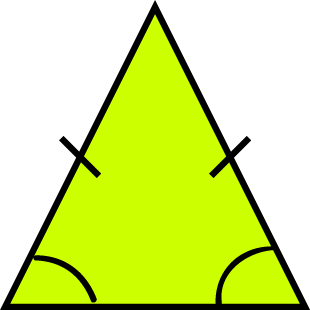# Definition of Isosceles TriangleAn isosceles triangle is a triangle in which two of the sides are equal in length.

The angles opposite the equal sides of an isosceles triangle (we call these the base angles) are also equal in size.

Note: You can equally well define an isosceles triangle to be a triangle with two equal angles. It will then follow that it has two equal sides. So, choose your definition, and remember it.

### Description

The aim of this dictionary is to provide definitions to common mathematical terms. Students learn a new math skill every week at school, sometimes just before they start a new skill, if they want to look at what a specific term means, this is where this dictionary will become handy and a go-to guide for a student.

### Audience

Year 1 to Year 12 students

### Learning Objectives

Learn common math terms starting with letter I

Author: Subject Coach
You must be logged in as Student to ask a Question.